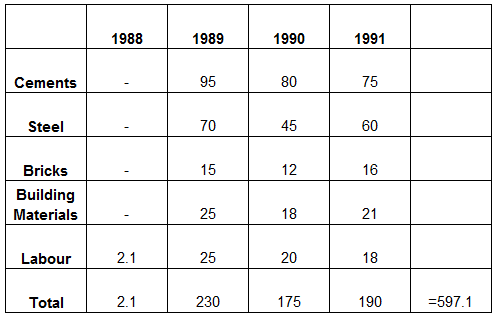### CAT 1990 Question Paper Question 62

Instructions

The table below shows the estimated cost (in Rs. Lakh) of a project of laying a railway line between two places.

Question 62

# If the length of line to be laid each year is in proportion to the estimated cost for material and labour, what fraction of the total length is proposed to be completed by the third year?

SolutionTill third year expense was 407.1 and total expenditure is 597.1

So ratio will be $$\frac{407.1}{597.1}$$ = 0.7
That will be fraction of length which is supposed to be completed by third year

• All Quant CAT Formulas and shortcuts PDF
• 30+ CAT previous papers with solutions PDF